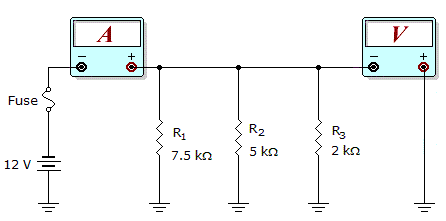# Electronics - Parallel Circuits - Discussion

Discussion Forum : Parallel Circuits - General Questions (Q.No. 11)
11.What would these meter readings indicate about the circuit in the given circuit?

Meter Readings: I = 7.6 mA, V = 12 V

R1 is open.
R2 is open.
The fuse is open.
The circuit is operating normally.
Explanation:
No answer description is available. Let's discuss.
Discussion:
17 comments Page 2 of 2.

Fantastic Himanshu Verma & Saichaitanya ..Great work.

Sriram pn said:   1 decade ago
Saichaitanya perfect explanation

@ Saichaitanya, Perfect explanation.

Well done Saichaitanya and Himanshu Verma.

I agree with Saichaithanya.

CONSIDER THE FOLLOWING:

In Parallel circuit, the current will differ and the voltage will remain same across all the branches. In other words, the voltage across R1, R2 and R3 will be 12V each.

I1= 12/7.5K => 1.6mA
I2= 12/5K => 2.4mA
I3= 12/2K => 6.0mA

In above, the total current 7.6mA will take place correctly when only I1 and I3 present *(since, I1 + I3 = 7.6mA).
Hence, R2 must be discarded/ remove from the circuit. In other words, R2 is open.
(1)

Himanshu Verma said:   1 decade ago
Case1 :When R1 is open,
then the effective resistance R = R2||R3
i.e. 5K||2K = 10/7 K
then current I = V/R = (12*7)/10K = 8.4 mA(Which is not correct ans i.e 7.6 mA)

Case2 : When R2 is open,
then the effective resistance R = R1||R3
i.e. 7.5K||2K = 30/19 K
then current I = V/R = (12*19)/30K = 7.6 mA(Which is d correct ans i.e 7.6 mA)

Case3: When the fuse is open,
then it is not possible to obtain voltage of 12 V

So the correct answer is option [b].